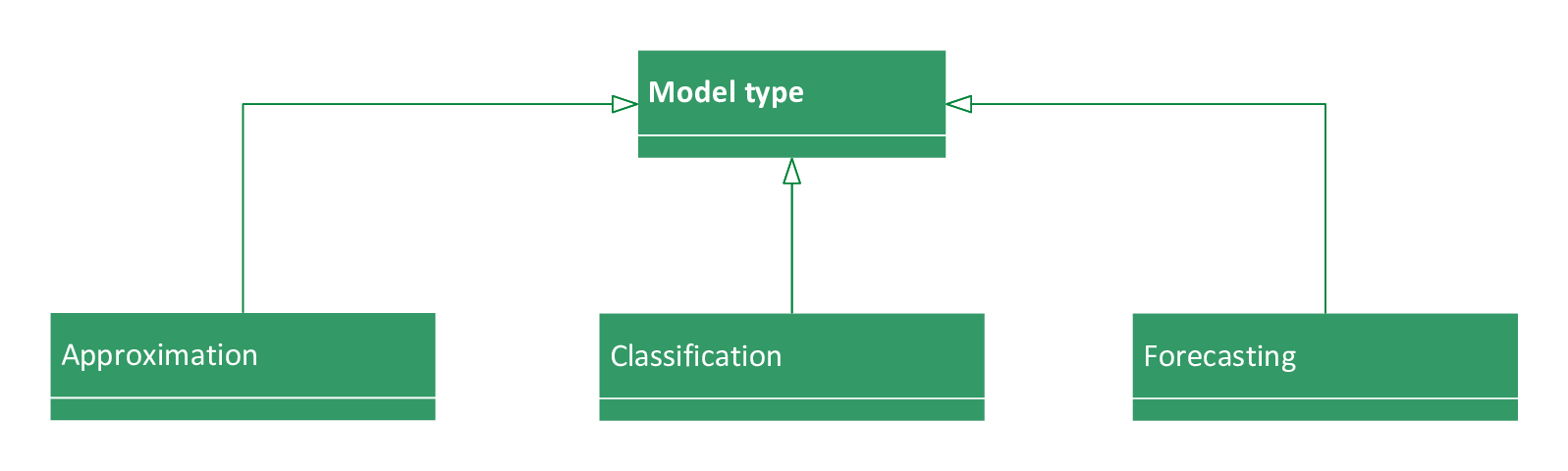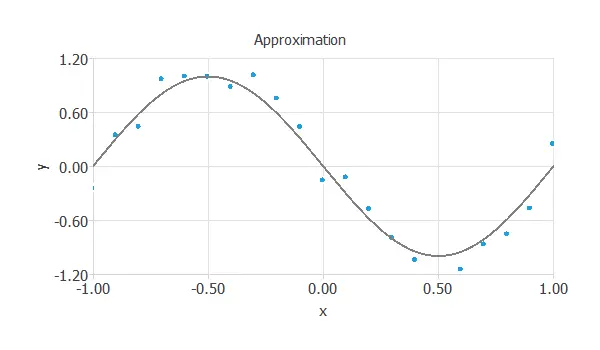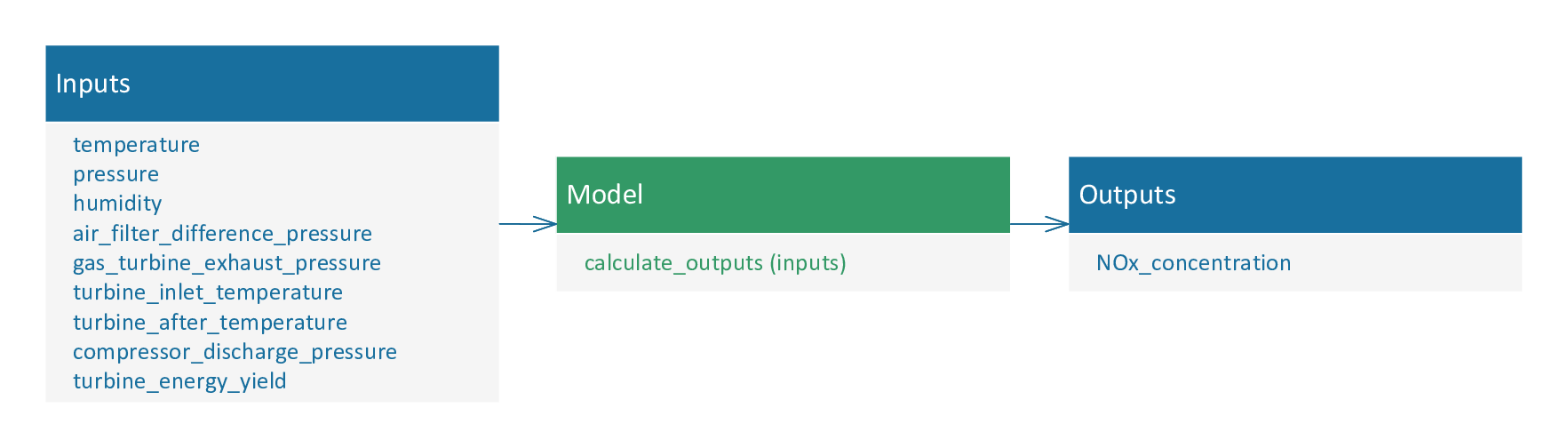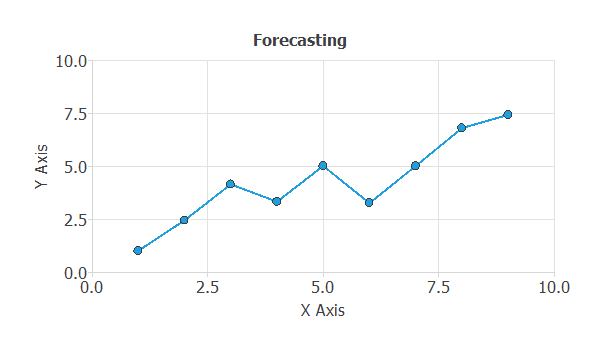## Introduction

A model describes a real-world system or process using mathematical concepts. They are used to make decisions or predictions in many fields and sectors, such as energy, medicine, marketing, etc.

In this regard, machine learning is a part of artificial intelligence that deals with building models without being explicitly programmed. In the machine learning field, a model is sometimes called a hypothesis.Supervised learning is the most common paradigm within machine learning. We can define it as the task of learning a function that maps an input to an output based on example input-output pairs.

A supervised learning algorithm analyses the training data and produces an inferred function, which can map new examples.
In supervised learning, each example is a pair consisting of an input object and the desired output value.

An optimal scenario will allow the algorithm to determine the class labels for unseen instances correctly. In this sense, generalization refers to the ability of a model to adapt correctly to new, previously unseen belonging from the same domain as that used to create the model.

The most important types of models in machine learning are approximation, classification, and forecasting. They allow us to discover relationships, recognize patterns, and predict trends from data.## 1. Approximation

An approximation model assigns a set of input variables to one or several output variables. Here the model learns from knowledge represented by a data set consisting of instances with input and target variables. The targets are a specification of the response to the inputs. In this regard, the primary goal of an approximation problem is to model one or several target variables, conditioned on the input variables.

The following figure illustrates an approximation problem. The objective here is to estimate the value of $y$, knowing the value of $x$. Figure ref{ApproximationFigure} illustrates a data set with $21$ samples, one input variable ($x$), and one target variable ($y$).It also shows the corresponding approximation model.

The following is an example of an approximation model in the industrial sector.

#### Reducing the gas emissions of a combined cycle power plant using machine learning

A combined cycle power plant that generates electricity wants to reduce its emissions of polluting gases, such as nitrogen oxides, which are harmful to health. The combined cycle power plant consists of gas turbines, steam turbines, and steam generators with heat recovery. Gaseous combustion generates electricity by driving steam turbines.

The power plant is sensorized and records all the data. From this, the company builds a model. The shape of this model is in the following figure:The input variables are atmospheric variables and plant-related variables. From them, the model is created, and the values of nitrogen oxide emissions produced by the plant are estimated.

## 2. Classification

A classification model assigns a set of features to one of a prescribed number of classes. We discuss binary classification if the classes are $true$ and $false$. An example is to diagnose a breast tumour as malignant ($true$) or being ($false$) from digitised images. We talk about multiple classifications when we want to discern among many classes.

In classification models, the targets are binary variables. Typical examples are customer segmentation or medical diagnosis.
Data sets for classification are very similar to that for approximation. The inputs here include features that characterise an object; the targets specify the corresponding class.

The fundamental goal in classification is to model the posterior probabilities of class membership, conditioned on the input variables. That means that the output of a classification model is the probability of an object belonging to each class.

The following image illustrates the case of a binary classification problem with two features:The central goal is to design a neural network with good generalisation capabilities. That is a model which can classify new data correctly.

The following is an example of an approximation model in the healthcare sector.

#### Early detection of lung carcinoma

Lung cancer is the leading cause of cancer death worldwide. This type of cancer does not show up in testing until later, after which treatments become ineffective or have lower success rates. For this reason, lung cancer must be diagnosed as early as possible.

To allow for early detection of lung cancer, a hospital builds a model that provides a tool to support lung cancer risk assessment and risk management to facilitate prevention and screening discussions between individuals and their physicians.

To build the model, a hospital creates a dataset containing lung cancer risk factors for $309$ patients.

The form of this model is in the following figure:The model estimates the probability that the patient will develop lung cancer from the clinical input variables. This will allow the hospital to closely follow new patients with a high probability of developing lung cancer.

## 3. Forecasting

A forecasting model makes predictions based on available information about the past.

The objective is to predict a system’s future state from past observations. An example is to forecast the future sales of a car model as a function of past sales, marketing actions, and macroeconomic variables.

The following figure illustrates a forecasting model:In forecasting applications, the data has a time-series format.

It consists of a sequence of variables observed at regular time intervals $tau=1,2,…$.

A forecasting problem can also be solved by approximating a function from input-target data.

Here, the inputs include past observations (predictors), and the targets include their corresponding future values (predictands).

As always, good generalisation is the central goal in forecasting applications.

We will now illustrate how prediction models work with an example.

#### Country’s inflation

A country’s government wants to predict next month’s inflation.

For this purpose, it uses a time series with macroeconomic variables.

A government uses data for the previous three months to predict a country’s inflation for the next month.

From these data, the following approximation model is created. The following figure shows the models form.Knowing the inflation and the consumer price index of the previous three months, the model is created, and the next month’s inflation is predicted.

This forecasting will allow the government to take corresponding measures as inflation rises or falls.

## Conclusions

The main machine learning models are approximation, classification, and prediction models.

A model uses mathematical concepts to describe a real-world system or process.

Good generalizability is the central objective when designing a model.